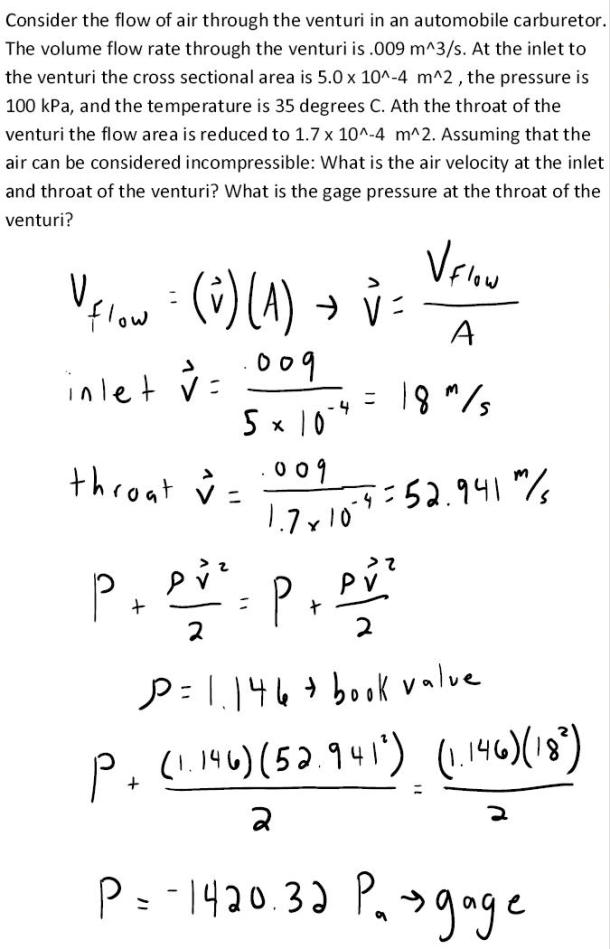fluid mechanics math problems engineering equations fluid mechanics basic mechanics school homework engineering math fluids formulas fluid mechanics problems fluid mechanics problem solutions to fluids problems full solution fluid mechanics engineering fluids problem solution fluid mechanics math problems engineering equations fluid mechanics basic mechanics school homework engineering math fluid mechanics formulas fluid problems fluid mechanics problem solutions to fluid mechanics problems full solution fluid mechanics engineering fluid mechanics problem solution
fluid mechanics math problems engineering equations fluid mechanics basic mechanics school homework engineering math fluids formulas fluid mechanics problems fluid mechanics problem solutions to fluids problems full solution fluid mechanics engineering fluids problem solution fluid mechanics math problems engineering equations fluid mechanics basic mechanics school homework engineering math fluid mechanics formulas fluid problems fluid mechanics problem solutions to fluid mechanics problems full solution fluid mechanics engineering fluid mechanics problem solution
Highalphabet Home Page fluid mechanics solutions fluids math solved Fluid Mechanics Page 1
Consider the flow of air through the venturi in an automobile carburetor. The volume flowrate through the venturi is .009 m^3/s. At the inlet to the venturi the cross sectional area is 5.0x10^-4 m^2, the pressure is 100 kPa, and the temperature is 35 degrees C. At the throat of the venturi the flow area is reduced to 1.7x10^-4 m^2. Assuming that the air can be considered incompressible: What is the air velocity at the inlet and throat of the venturi? What is the gage pressure at the throat of the venturi?Consider the flow of air through the venturi in an automobile carburetor. The volume flowrate through the venturi is .009 m^3/s. At the inlet to the venturi the cross sectional area is 5.0x10^-4 m^2, the pressure is 100 kPa, and the temperature is 35 degrees C. At the throat of the venturi the flow area is reduced to 1.7x10^-4 m^2. Assuming that the air can be considered incompressible: What is the air velocity at the inlet and throat of the venturi? What is the gage pressure at the throat of the venturi?